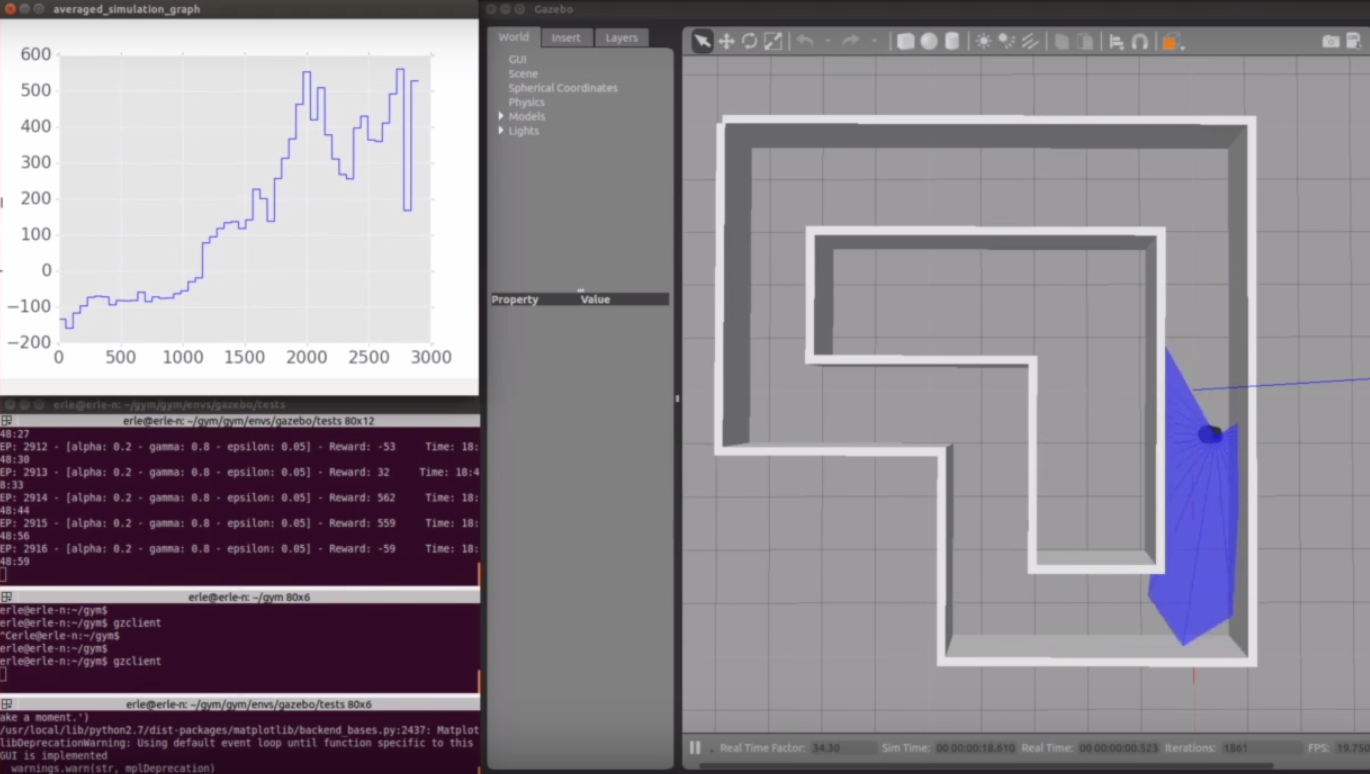Content based on Erle Robotics's whitepaper: Extending the OpenAI Gym for robotics: a toolkit for reinforcement learning using ROS and Gazebo.

The OpenAI Gym is a is a toolkit for reinforcement learning research that has recently gained popularity in the machine learning community. The work presented here follows the same baseline structure displayed by researchers in the OpenAI Gym, and builds a gazebo environment on top of that. OpenAI Gym focuses on the episodic setting of RL, aiming to maximize the expectation of total reward each episode and to get an acceptable level of performance as fast as possible. This toolkit aims to integrate the Gym API with robotic hardware, validating reinforcement learning algorithms in real environments. Real-world operation is achieved combining Gazebo simulator, a 3D modeling and rendering tool, with the Robot Operating System, a set of libraries and tools that help software developers create robot applications.

As discussed previously, the main problem with RL in robotics is the high cost per trial, which is not only the economical cost but also the long time needed to perform learning operations. Another known issue is that learning with a real robot in a real environment can be dangerous, specially with flying robots like quad-copters. In order to overcome this difficulties, advanced robotics simulators like Gazebo have been developed which help saving costs, reducing time and speeding up the simulation.

## Architecture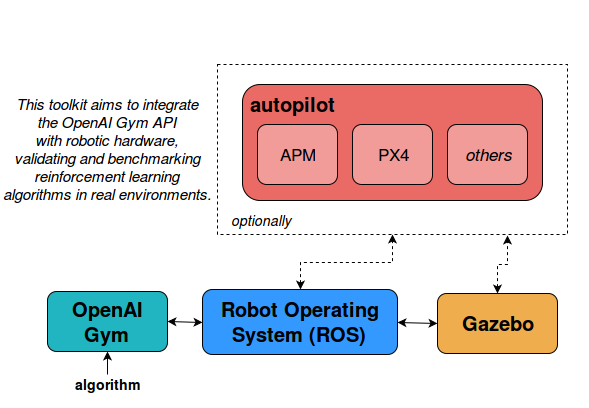Simplified software architecture used in OpenAI Gym for robotics.

The architecture consists of three main software blocks: OpenAI Gym, ROS and Gazebo. Environments developed in OpenAI Gym interact with the Robot Operating System, which is the connection between the Gym itself and Gazebo simulator. Gazebo provides a robust physics engine, high-quality graphics, and convenient programmatic and graphical interfaces.

The architecture described was tested with three different robots: - Erle-Copter - Erle-Rover - Turtlebot

It's relevant to note that within the architecture proposed, our team considered also robots with an autopilot. This optional element, common in certain environments adds a layer of complexity to the overall simulation setup.

## Getting your robot into the gym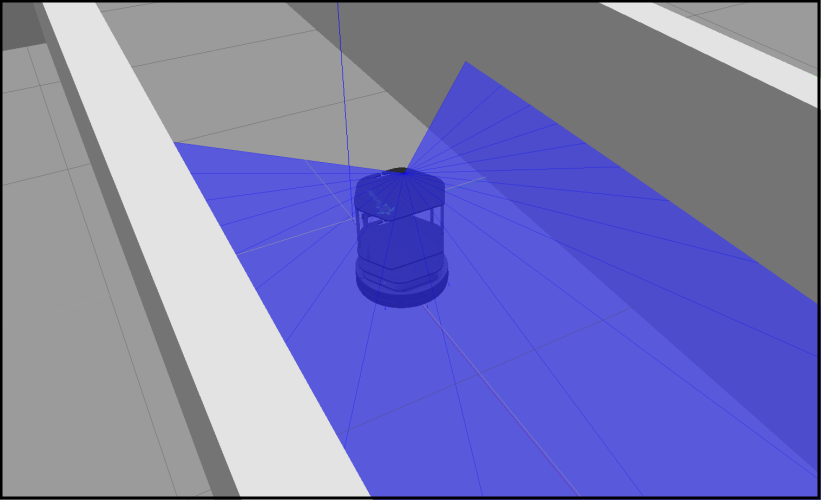Let’s go ahead and code of a simple example with this OpenAI Gym extension for robotics (that we call the robot gym). We’ll take the Turtlebot and use Reinforcement Learning (Q-Learning particularly) to teach the robot how to avoid obstacles using only a simulated LIDAR:

Getting everything ready for the robot gym to work will need you to set it up appropriately. Refer to these instructions and do it yourself.

If you're looking for full and complete code example, refer to circuit2_turtlebot_lidar_qlearn.py.

First, we define a QLearn class that will be used later in our gym script:

import random

class QLearn:
def __init__(self, actions, epsilon, alpha, gamma):
self.q = {}
self.epsilon = epsilon  # exploration constant
self.alpha = alpha      # discount constant
self.gamma = gamma      # discount factor
self.actions = actions

def getQ(self, state, action):
return self.q.get((state, action), 0.0)

def learnQ(self, state, action, reward, value):
'''
Q-learning:
Q(s, a) += alpha * (reward(s,a) + max(Q(s') - Q(s,a))
'''
oldv = self.q.get((state, action), None)
if oldv is None:
self.q[(state, action)] = reward
else:
self.q[(state, action)] = oldv + self.alpha * (value - oldv)

def chooseAction(self, state, return_q=False):
q = [self.getQ(state, a) for a in self.actions]
maxQ = max(q)

if random.random() < self.epsilon:
minQ = min(q); mag = max(abs(minQ), abs(maxQ))
# add random values to all the actions, recalculate maxQ
q = [q[i] + random.random() * mag - .5 * mag for i in range(len(self.actions))]
maxQ = max(q)

count = q.count(maxQ)
# In case there're several state-action max values
# we select a random one among them
if count > 1:
best = [i for i in range(len(self.actions)) if q[i] == maxQ]
i = random.choice(best)
else:
i = q.index(maxQ)

action = self.actions[i]
if return_q: # if they want it, give it!
return action, q
return action

def learn(self, state1, action1, reward, state2):
maxqnew = max([self.getQ(state2, a) for a in self.actions])
self.learnQ(state1, action1, reward, reward + self.gamma*maxqnew)


Now, the real fun. We just need to import the corresponding environment and call the traditional OpenAI gym’s primitives (env.reset(), env.step(action), etc.):

env = gym.make('GazeboCircuit2TurtlebotLidar-v0')

outdir = '/tmp/gazebo_gym_experiments'
env.monitor.start(outdir, force=True, seed=None)
last_time_steps = numpy.ndarray(0)
qlearn = qlearn.QLearn(actions=range(env.action_space.n),
alpha=0.2, gamma=0.8, epsilon=0.9)

initial_epsilon = qlearn.epsilon
epsilon_discount = 0.9986
start_time = time.time()
total_episodes = 10000
highest_reward = 0

for x in range(total_episodes):
done = False
cumulated_reward = 0
observation = env.reset()
if qlearn.epsilon > 0.05:
qlearn.epsilon *= epsilon_discount
state = ''.join(map(str, observation))

for i in range(1500):
# Pick an action based on the current state
action = qlearn.chooseAction(state)

# Execute the action and get feedback
observation, reward, done, info = env.step(action)
cumulated_reward += reward

if highest_reward < cumulated_reward:
highest_reward = cumulated_reward

nextState = ''.join(map(str, observation))

qlearn.learn(state, action, reward, nextState)
if not(done):
state = nextState
else:
last_time_steps = numpy.append(last_time_steps, [int(i + 1)])
break

m, s = divmod(int(time.time() - start_time), 60)
h, m = divmod(m, 60)
print "EP: "+str(x+1)+" - [alpha: "+str(round(qlearn.alpha,2))+" - gamma: "+str(round(qlearn.gamma,2))+" - epsilon: "+str(round(qlearn.epsilon,2))+"] - Reward: "+str(cumulated_reward)+"     Time: %d:%02d:%02d" % (h, m, s)

env.monitor.close()
env.close()



By default, you’ll see that the environment gets launched without a graphical interface but you can bring it up by typing gzclient into a new command line prompt.

## Results

Currently, plotting tools are not completely integrated within the gym APIs (e.g.: env.monitor could be further improved in the Gazebo abstraction) however it’s possible to get life plots of the robot performance like the one that follows: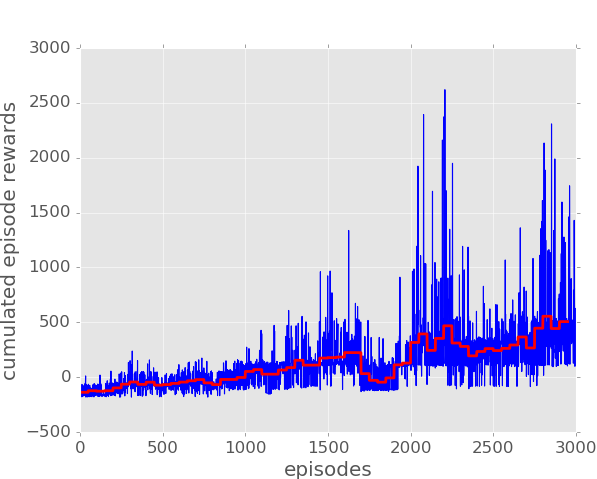Results of the Turtlebot robot while learning how to avoid obstacles in the gym after 3000 iterations running a Q-Learning algorithm.

While not a universal benchmarking mechanism, the overall architecture proposed serves its purpose when it comes to benchmarking different techniques. The plot below displays the same robot using the SARSA algorithm instead: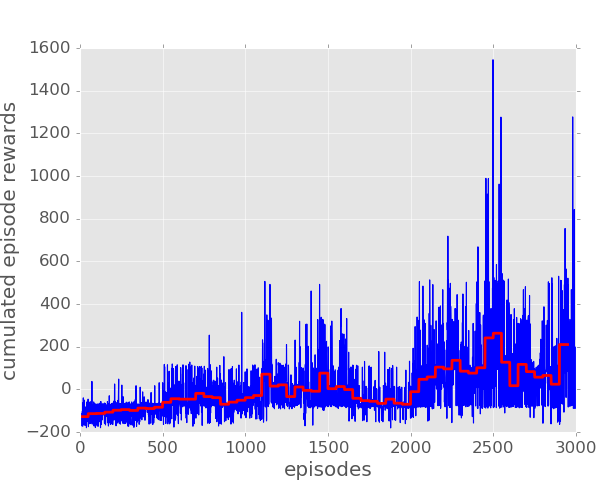Results of the Turtlebot robot while learning how to avoid obstacles in the gym after 3000 iterations running a Sarsa algorithm.

Comparing results one can tell that in this particular scenario, the learning in Q-Learning occurs faster than in Sarsa, this happens because Q-Learning is able to learn a policy even if taken actions are chosen randomly. However, Q-Learning shows more risky moves (taking turns really close to walls) while in Sarsa we see a smoother general behaviour. The major difference between Sarsa and Q-Learning, is that the maximum reward for the next state is not necessarily used for updating the Q-values (learning table). Instead, a new action, and therefore reward, is selected using the same policy that determined the original action. This is how Sarsa is able to take into account the control policy of the agent during learning. It means that information needs to be stored longer before the action values can be updated, but also means that our robot is going to take risky actions much frequently.

This smoother behaviour where forward actions are being exploited in straight tracks leads to higher maximum cumulated rewards. We get values near 3500 in Sarsa while just get cumulated rewards around 2500 in Q-Learning. Running Sarsa for more episodes will cause to get higher average rewards.

## Resources

• Extending the OpenAI Gym for robotics: a toolkit for reinforcement learning using ROS and Gazebo whitepaper (pdf)
• Erle Robotics gym fork
• Brockman, G., Cheung, V., Pettersson, L., Schneider, J., Schulman, J., Tang, J., & Zaremba, W. (2016). OpenAI Gym. arXiv preprint arXiv:1606.01540.